# Plotting the fitted models

#### 2019-08-22

The sicegar package provides a helpful function that can generate plots of the fitted models. This function is built on top of the ggplot2 plot library.

To demonstrate figure generation, we first generate simulated sigmoidal and double-sigmoidal data and fit the respective models to these datasets.

# simulate sigmoidal data
time <- seq(3, 24, 0.5)

noise_parameter <- 0.1
intensity_noise <- runif(n = length(time), min = 0, max = 1) * noise_parameter
intensity <- sigmoidalFitFormula(time, maximum = 4, slope = 1, midPoint = 8)
intensity <- intensity + intensity_noise
dataInputSigmoidal <- data.frame(intensity = intensity, time = time)

# simulate double-sigmoidal data
noise_parameter <- 0.2
intensity_noise <- runif(n = length(time),min = 0,max = 1) * noise_parameter
intensity <- doublesigmoidalFitFormula(time,
finalAsymptoteIntensityRatio = .3,
maximum = 4,
slope1 = 1,
midPoint1Param = 7,
slope2 = 1,
midPointDistanceParam = 8)
intensity <- intensity + intensity_noise
dataInputDoubleSigmoidal <- data.frame(intensity = intensity, time = time)

# fit models to both datasets
fitObj_sm <- fitAndCategorize(dataInput = dataInputSigmoidal)
fitObj_dsm <- fitAndCategorize(dataInput = dataInputDoubleSigmoidal)

Now we can plot the results using the function figureModelCurves(). This function returns a ggplot2 plot that can be saved or displayed directly. The function has several different options.

First, we can plot only the raw input data.

# sigmoidal raw data only
figureModelCurves(dataInput = fitObj_sm$normalizedInput)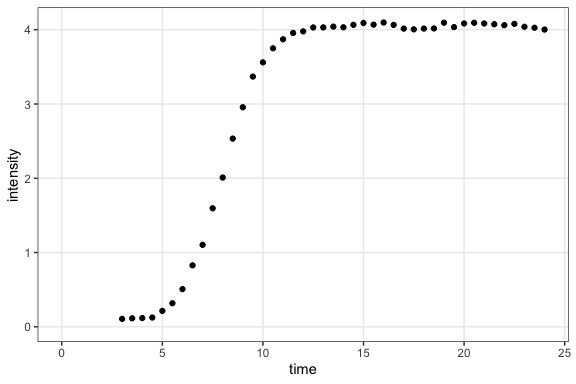# double-sigmoidal raw data only figureModelCurves(dataInput = fitObj_dsm$normalizedInput)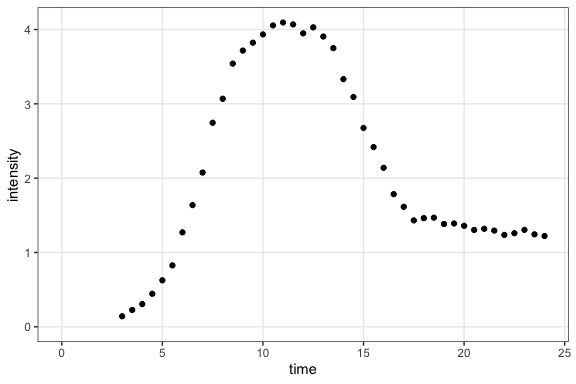Second, we can plot the input data with the fitted lines.

# sigmoidal fit
figureModelCurves(dataInput = fitObj_sm$normalizedInput, sigmoidalFitVector = fitObj_sm$sigmoidalModel)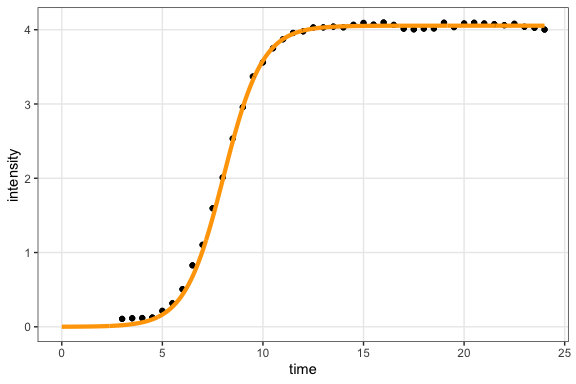# double-sigmoidal fit
figureModelCurves(dataInput = fitObj_dsm$normalizedInput, doubleSigmoidalFitVector = fitObj_dsm$doubleSigmoidalModel)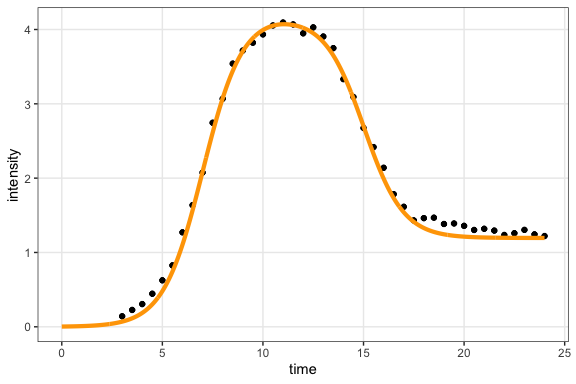Third, we can additionally visualize the parameter estimates, by setting showParameterRelatedLines = TRUE.

# sigmoidal fit with parameter related lines
figureModelCurves(dataInput = fitObj_sm$normalizedInput, sigmoidalFitVector = fitObj_sm$sigmoidalModel,
showParameterRelatedLines = TRUE)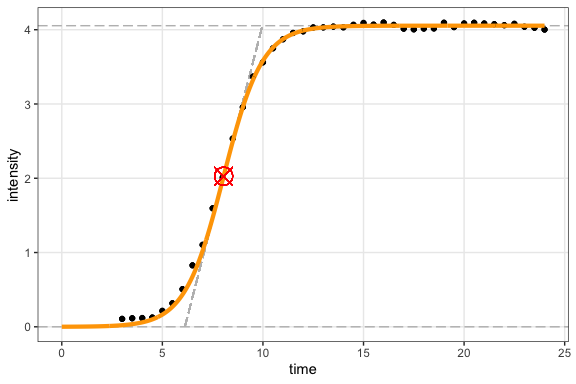# double-sigmoidal fit with parameter related lines
figureModelCurves(dataInput = fitObj_dsm$normalizedInput, doubleSigmoidalFitVector = fitObj_dsm$doubleSigmoidalModel,
showParameterRelatedLines = TRUE)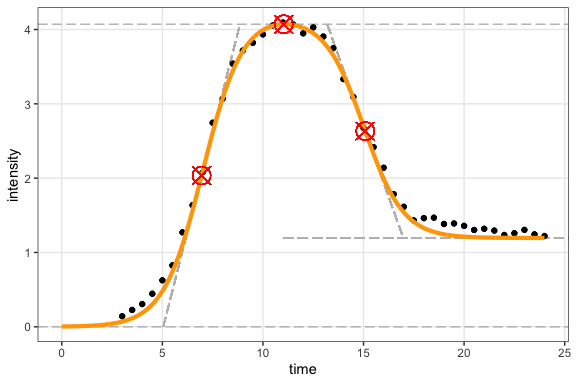Note that the last example only works for models that had additional parameters calculated using parameterCalculation(). This is done automatically when fitting with fitFunction(), but needs to be done manually when fitting with multipleFitFunction().